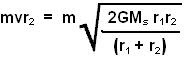## Sunday, September 7, 2008

### Irodov Problem 1.207

In the solution of problem 1.202 equation 6, we derived for the closest approach of the planet that,Here r2 is the closest approach of the planet to the sun, r1 is the farthest approach to the sun, v is the tangential velocity of the planet at its closet approach to the sun, RA is the radius of curvature of the path of the planet at its closest approach to the sun, and Ms is the mass of the sun. Please refer to the solution for problem 1.202 to find out how to arrive at this result.

The angular momentum then simply is given by,